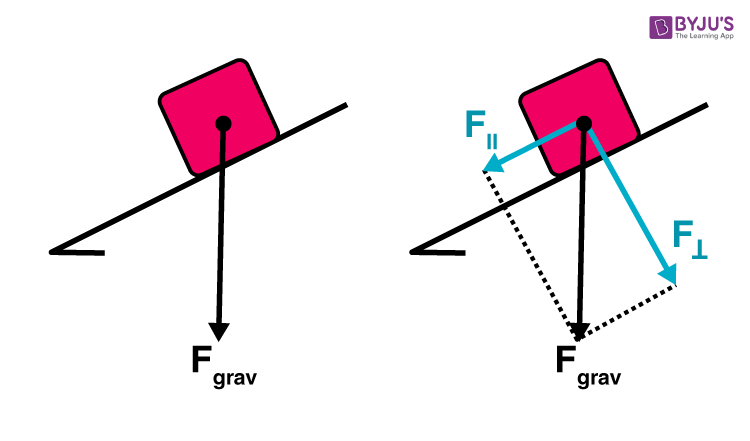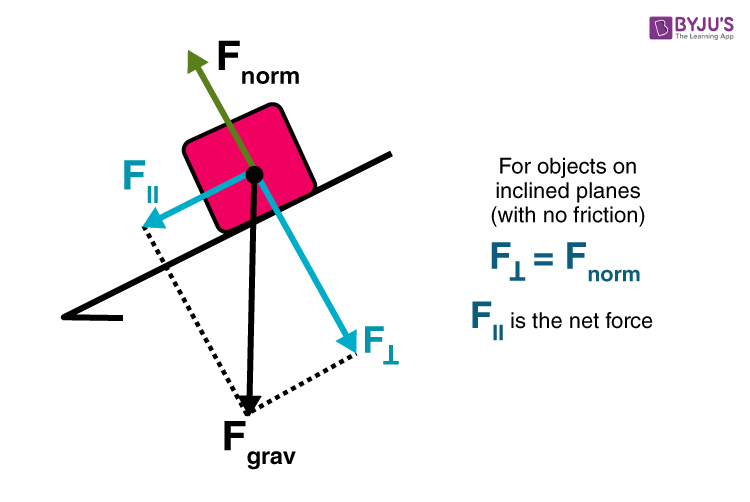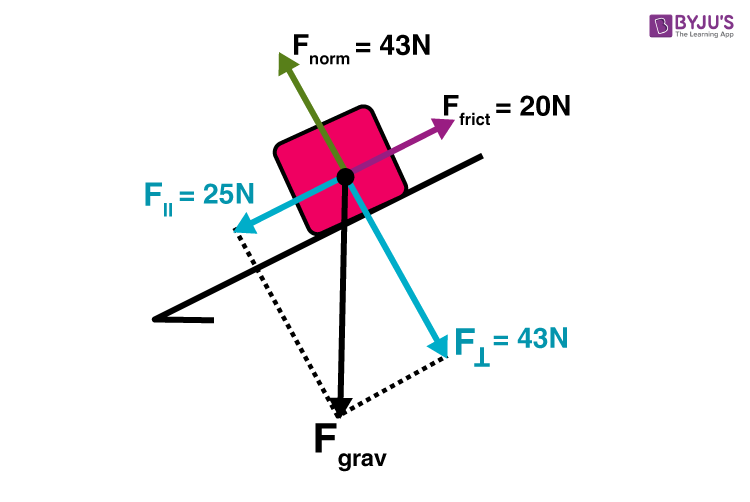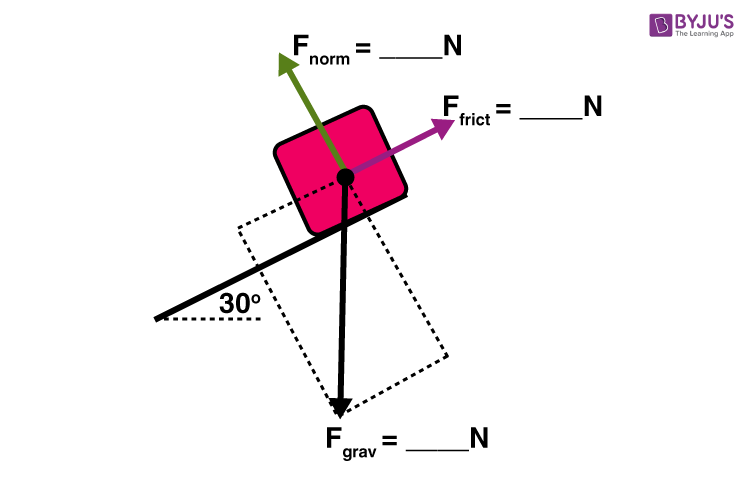# Inclined Planes

An inclined plane, also known as a ramp, is a flat supporting surface tilted at an angle, with one end higher than the other. The inclined plane is one of the six simple machines, and it is used as an aid for raising or lowering a load. Objects placed on an inclined plane accelerate due to an unbalanced force. In this article, let us examine the motion exhibited by an object placed on an inclined plane.

## Normal Force in Inclined Planes

The normal force in an inclined plane is not directed in the direction that we are accustomed to. Up until now, we have always seen normal force directed upwards in the direction opposite to the force of gravity. The truth about normal forces is that they are not always upwards but rather that they are always directed perpendicular to the surface that the object is on.

### Gravity Force Components

To determine the net force acting upon an object on an inclined plane is difficult because the two forces acting on the body are not in opposite directions. To simplify, one of the forces acting on the object will have to be resolved into perpendicular components so that they can be easily added to other forces acting on the object. The force directed at an angle to the horizontal is resolved into horizontal and vertical components.

In the case of inclined planes, we resolve the weight vector (Fgrav) into two components. The force of gravity will be resolved into two components of force – one directed parallel to the inclined surface and the other directed perpendicular to the inclined surface. The diagram below shows how the force of gravity has been replaced by two components – a parallel and a perpendicular component of force.From the figure, we understand that the perpendicular component of the force of gravity is directed in the opposite direction of the normal force, balancing the normal force. Any other force does not balance the parallel component of the force of gravity. The object will subsequently accelerate down the inclined plane due to the presence of an unbalanced force. The parallel component of the force of gravity causes this acceleration. The parallel component of the force of gravity is the net force.

The equations used to determine the magnitude of the two components of the force of gravity are$$\begin{array}{l}F_\parallel =mg\sin \Theta\end{array}$$

$$\begin{array}{l}F_\perp=mg\cos\Theta\end{array}$$

In the absence of friction and other forces, the acceleration of an object is the value of the parallel component divided by the mass. This yields the equation,

$$\begin{array}{l}a=g\sin \Theta\end{array}$$

## Recommended Videos

#### Projectile Motion on an Inclined Plane>

#### Friction on an Incline## Solving an Inclined Plane Problem

In the presence of friction and other forces, such as applied force and tensional force, it gets slightly complicated.In the given diagram, the perpendicular component of the force balances the normal force. To find the net force, all the forces must be added. The perpendicular component and the normal force sum up to 0 N. The parallel component and the friction force add together to yield 5 N. The net force is 5 N, directed along the incline towards the floor.

Let us consider another example.The free-body diagram shows the forces acting upon a 100-kg crate sliding down an inclined plane. The plane is inclined at an angle of 30 degrees. The coefficient of friction between the crate and the incline is 0.3. Determine the net force and acceleration of the crate.

Solution:

The force of gravity in the given problem can be calculated as:

F = 9.8 * 100 = 980 N

The components of the force of gravity can be determined as follows:

Fparallel = (980 * sin30) = 490 N

Fperpendicular = (980 * cos30) = 849 N

As the perpendicular component of the weight vector balances the normal force, the value of the weight vector is 849 N

The value of the frictional force can be determined by multiplying the value of normal force and the coefficient of friction.

Fnorm = 0.3*849 =255 N

The net force is the vector sum of all the forces acting on the body.

The net force can be calculated as follows:

490 N – 255 N = 235 N

The acceleration is calculated as follows:

Fnet/m = 235 N/100 kg = 2.35 m/s2

 Similar Articles:

## Frequently Asked Questions – FAQs

Q1

### What is an Inclined Plane?

An inclined plane is a flat supporting surface tilted at an angle, with one end higher than the other.
Q2

### Why do we use Inclined Planes?

An inclined plane is used as an aid for raising or lowering a load.
Q3

### Is an inclined plane a simple machine?

An inclined plane s one of the six simple machines.
Q4

### Why do objects placed in an inclined plane accelerate?

Objects placed in an inclined plane accelerate due to unbalanced forces.
Q5

### How does friction affect inclined planes?

In the case of an incline, the force of gravity is not perpendicular to the surface. As the angle of the incline increases, the normal force decreases, which decreases the frictional force. The incline can be raised until the object just begins to slide.

Like an incline, a lever is a simple machine that helps us lift heavy loads. But how does it achieve this? What are the parts of a lever? How do they work? Watch the video to find answers to all your questions.Stay tuned to BYJU’S and FALL IN LOVE WITH LEARNING!

Test your knowledge on Acceleration Inclined Plane